# Algebra 1 : How to find the solution to an inequality with addition

## Example Questions

1 2 4 Next →

### Example Question #80 : Equations / Inequalities

Solve the inequality: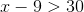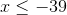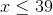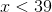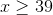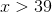Explanation:

In order to find the solution to this inequality, add nine on both sides.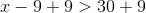Simplify both sides.  There is no need to change the direction of the sign.

The answer is:### Example Question #81 : Equations / Inequalities

Solve the inequality: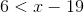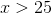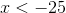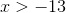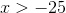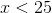Explanation:

Solve by adding nineteen on both sides.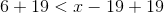Simplify both sides.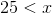This is also the same as:The answer is:### Example Question #82 : Equations / Inequalities

Solve the inequality: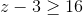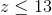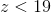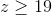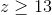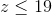Explanation:

In order to solve this inequality, add three on both sides.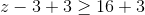Simplify both sides of the inequality.

The answer is:### Example Question #83 : Equations / Inequalities

Solve the following inequality: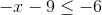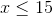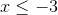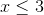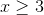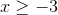Explanation: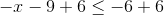Simplify both sides.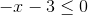Addon both sides.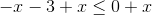Simplify the left side.  Since we are adding a negative variable, it is not necessary to change the sign.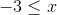The answer is:### Example Question #84 : Equations / Inequalities

Solve the inequality: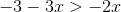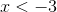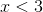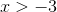Explanation:

Group the x-variables by addingon both sides of the equation.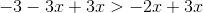Simplify both sides of the equation.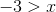Since we did not divide by a negative number, we do not need to switch the direction of the sign.

The answer is:### Example Question #85 : Equations / Inequalities

Solve the inequality: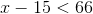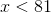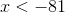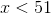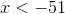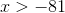Explanation:

In order to isolate the x-variable, add 15 on both sides of equation.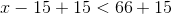Simplify both sides of the inequality.

The answer is:1 2 4 Next →